•   Excel 数据验证也叫数据有效性，它可以控制单元格内输入的内容，让数据的输入更加准确、规范，的确为表单制作的一个神器。本文除了介绍常用功能外，还列举了一堆自定义的函数供你验证数据。 一、常用功能 验证...
  Excel 数据验证也叫数据有效性，它可以控制单元格内输入的内容，让数据的输入更加准确、规范，的确为表单制作的一个神器。本文除了介绍常用功能外，还列举了一堆自定义的函数供你验证数据。
一、常用功能
验证数据的我们常用的功能是 “序列” ，此外你还可以限制在指定的单元格中只能输入整数、数值，日期、时间，或者限制输入文本的长度。
1） “序列” ，例如限制只能输入是和否（左图），选择性别（右图）。如下：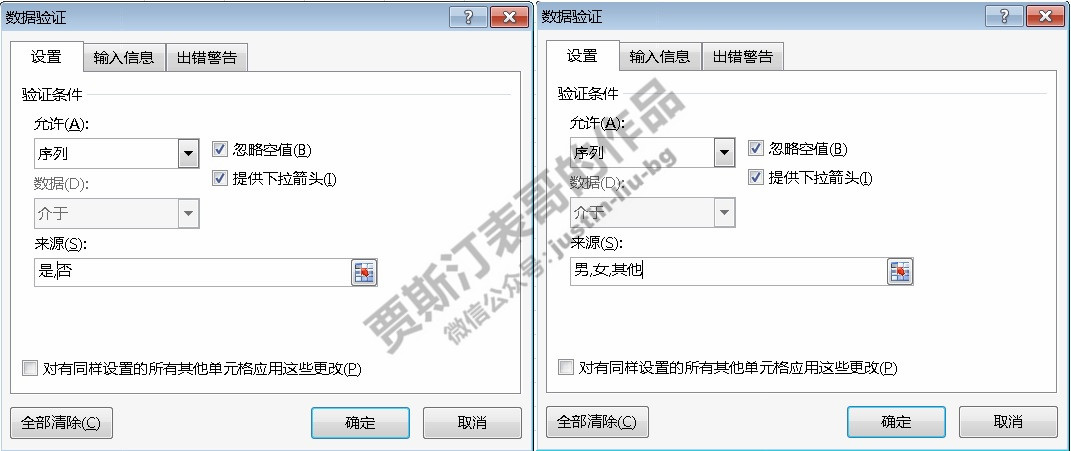只能输入指定单元格内的数据，例如只能输入$A$2 到 $A$16的部门、或产品信息，如下图：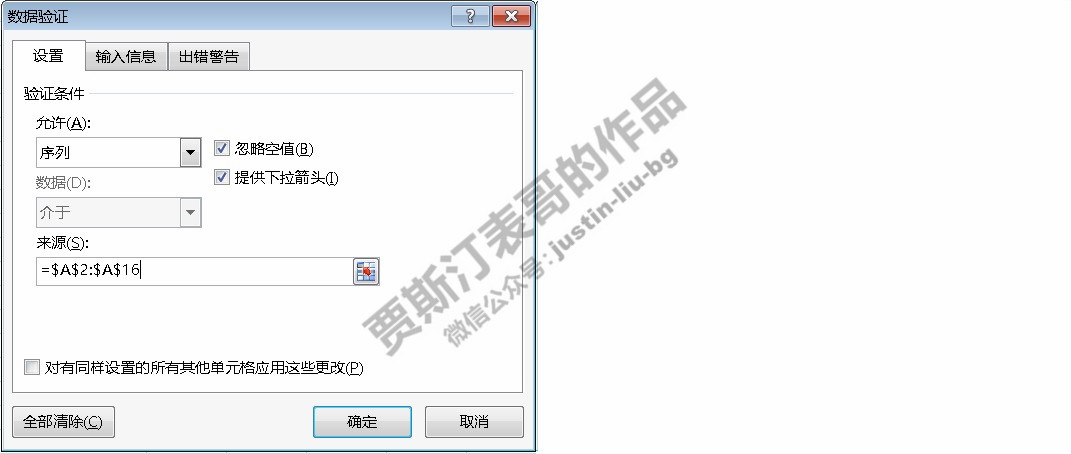2) 只能输入介于50到1000的整数（左图）、大于或者等于零的数值（右图）：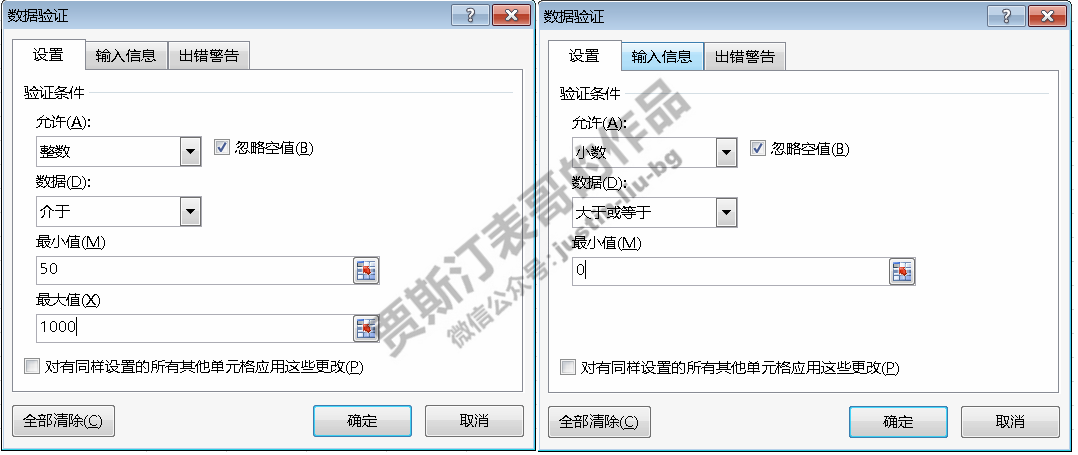3) 只能输入日期（左图）或时间（右图）：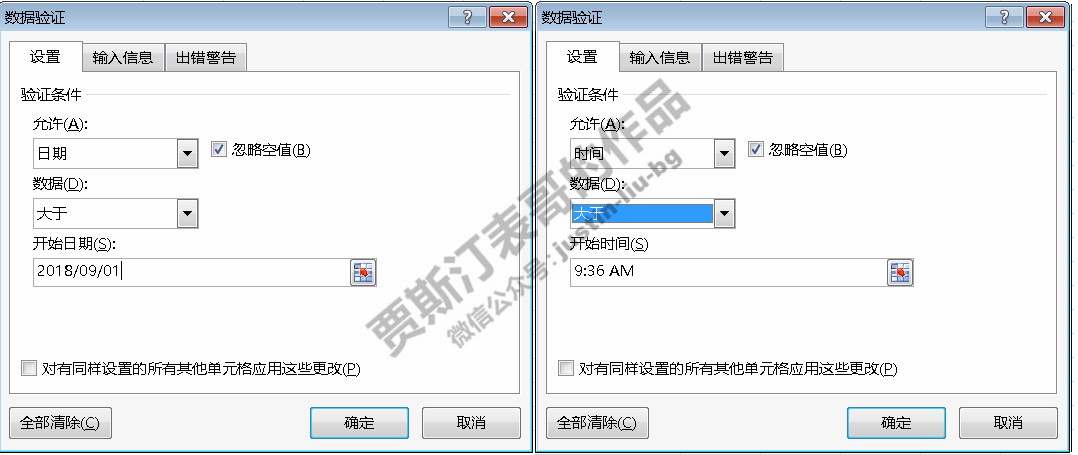4）限制输入的文本长度。
二、自定义公式限制输入内容
例如在 A 列不能输入重复值（左图），单元格只接受输入文本（右图）：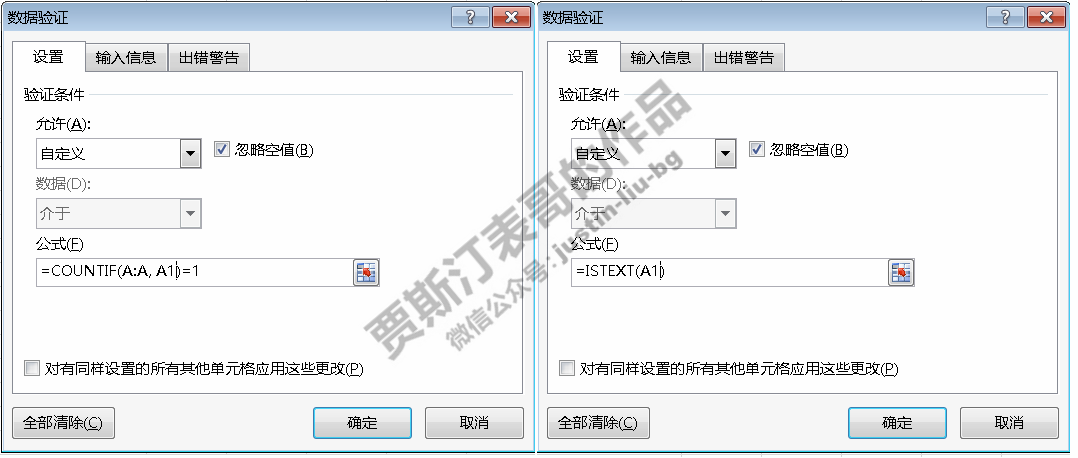其他常用的公式还有：
不允许输入空格字符：= LEN(A1) = LEN(SUBSTITUTE(A1, " ", “”))  只接受大写字符：= EXACT(A1, UPPER(A1))  只接受小写字符：= EXACT(A1, LOWER(A1))  必须依字符串 PRO 开头：= LEFT(A1, 3) = “PRO”  只有在 B 列 和 C 列填写完后才能填写：= AND(B1 <> “”, C1 <> “”)  只接受 YYMMDD：= TEXT(DATE(LEFT(A1, 2), MID(A1, 3, 2), RIGHT(A1, 2)), “YYMMDD”) = TRIM(A1)  只接受财年：= AND(LEFT(A1, 2) = “FY”, ISNUMBER(INT(RIGHT(A1, 2))))  输入手机号码：= AND(INT(LEFT(A1, 3)) < 200, LEN(A1)=11, ISNUMBER(INT(A1)))  只能录入文本：=ISTEXT(A1)  严禁修改 = ISBLANK(B3:C9)。
欢迎评论，如果你喜欢这篇文章, 请记得点赞哦，谢谢！
本文为作者原创文章，未经作者允许不得转载。展开全文数据验证 函数
• 利用Excel数据有效性功能，可以定制下拉列表框，提高输入效率。 　在使用Excel的过程中，经常需要录入大量的数据，有些重复输入的数据往往还要注意数据格式等有效性。如果每个数据都通过键盘来输入，不仅浪费时间...
• (1)在I1单元格加入有效性检查 菜单 “数据” -> “有效性” -> “设置” ->...菜单 “数据” -> “有效性” -> “设置” -> “允许” -> “自定义” -> “公式” -> “=OR(AND(I1="无",J1=""),AND(I1="有",OR(J1="是",
=IF($I1="无",($J2="")*($K2="")) 意思就是，我I列输入是“无”的话，J列和K列就为空。 然后我需要完善一下这个公式，要求：我I列输入是“无”的话，J列和K列就为空，或者 列输入是“有”的情况，列和K列只能输入“是”或者“否”。 (1)在I1单元格加入有效性检查 菜单 “数据” -> “有效性” -> “设置” -> “允许” -> “序列” -> “来源” -> “无,有” -> “确定” (2)在J1单元格加入有效性检查 菜单 “数据” -> “有效性” -> “设置” -> “允许” -> “自定义” -> “公式” -> “=OR(AND(I1="无",J1=""),AND(I1="有",OR(J1="是",J1="否")))” (3)在K1单元格加入有效性检查 菜单 “数据” -> “有效性” -> “设置” -> “允许” -> “自定义” -> “公式” -> “=OR(AND(I1="无",K1=""),AND(I1="有",OR(K1="是",K1="否")))” (4)选中整个J列，菜单 “数据” -> “有效性” -> “对话框提示” -> “是” (5)选中整个J列，菜单 “数据” -> “有效性” -> “对话框提示” -> “是” 上面的方案只是限制了I列和J列不能乱输入(乱输会报错)，但没有限制I列选定后乱改，由于是公式方案，也没有办法做到“不能保存”。  展开全文• NULL 博文链接：https://skycb.iteye.com/blog/2363483 • c#npoi获取数据有效性序列下拉框的值，之前代码没保存。。 • 方法一：引用名称法 1、在Sheet中创建“1-8”的序列；...4、打开Sheet2页，数据有效性添加一个序列，数据来源直接填入“=数据”； 5、设置成功。 方法二：公式法 1、在方法一的步骤2中可以，直接查看到公... 方法一：引用名称法 1、在Sheet中创建“1-8”的序列；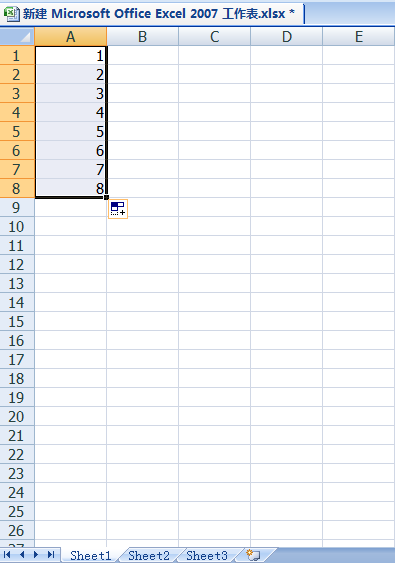2、公式—定义名称，创建一个名为“数据”的名称；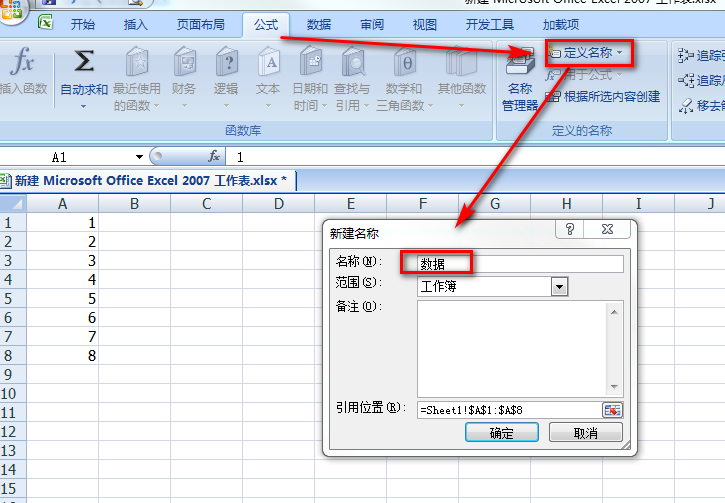3、在名称栏，被选中的单元格，显示名称为“数据”；（直接选中单元格，然后在名称栏直接进行修改更加便捷）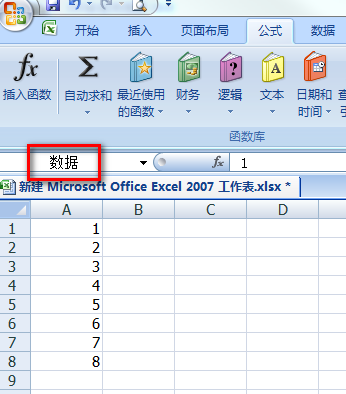4、打开Sheet2页，数据有效性添加一个序列，数据来源直接填入“=数据”；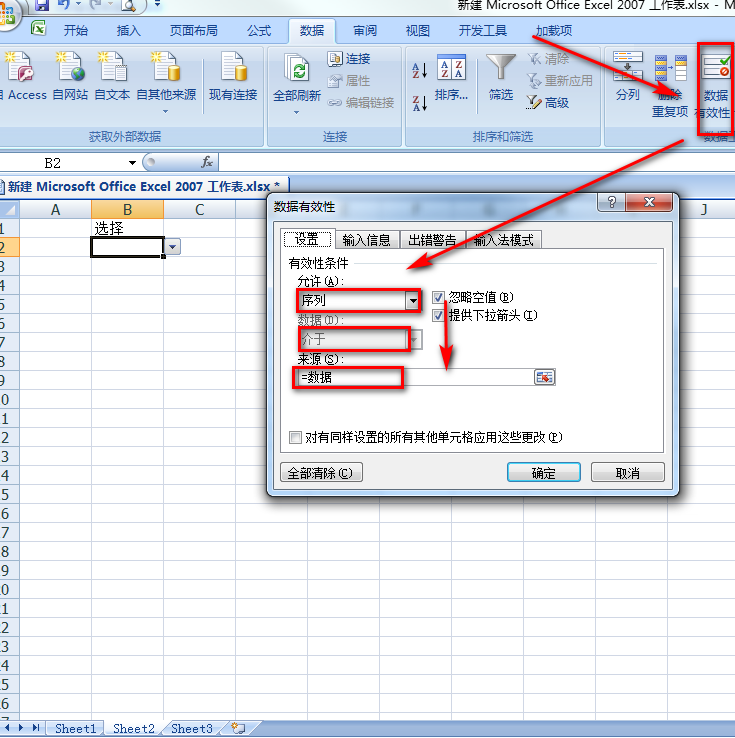5、设置成功。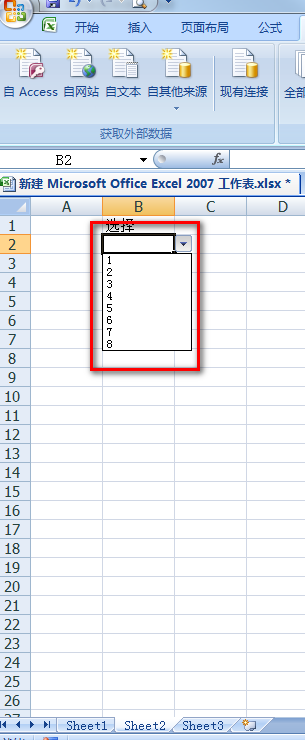方法二：公式法 1、在方法一的步骤2中可以，直接查看到公式（引用位置）“=Sheet1!$A$1:$A$8”（也可以“=Sheet1!A1:A8”）， ps：当然如果对于公式熟悉可以直接用。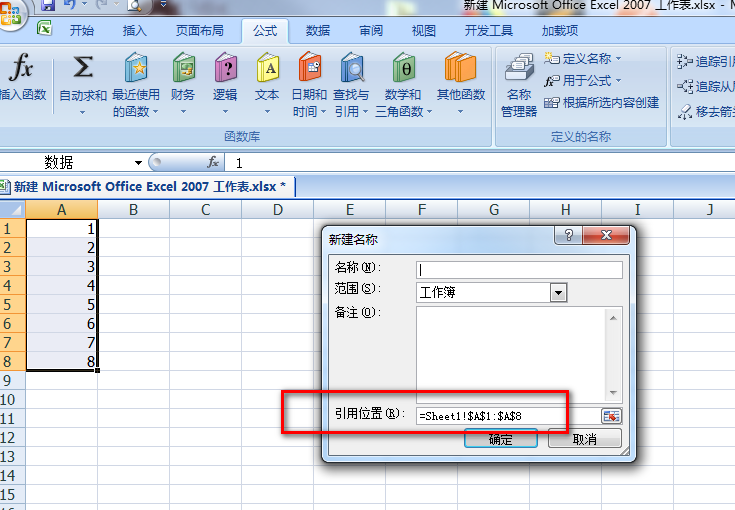2、数据有效性中，添加序列，来源为“=Sheet1!$A$1:$A$8”；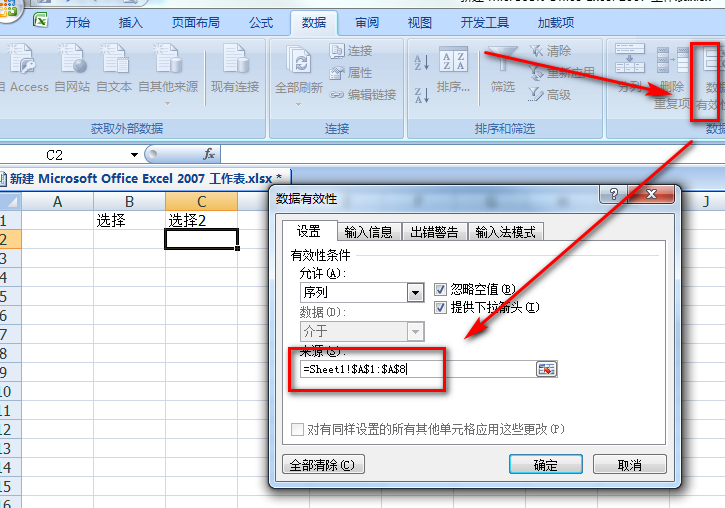3、设置成功。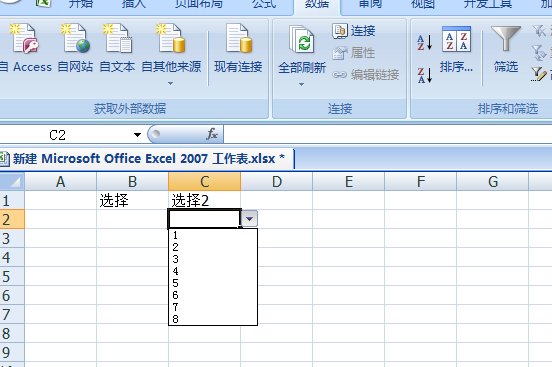展开全文• Excel数据有效性功能，可以对输入单元格的数据进行内容上的限制。当我们利用 excel 生成代码时，可以通过此类方式规范输入。 以下是：【openpyxl官方文档】 excel的基本操作 生成xlsx wb = Workbook() wb.save... Excel的数据有效性功能，可以对输入单元格的数据进行内容上的限制。当我们利用 excel 生成代码时，可以通过此类方式规范输入。 以下是：【openpyxl官方文档】 excel的基本操作 生成xlsx wb = Workbook() wb.save(xls_path)  加载excel文件 # 加载excel文件(data_only=True 代表以数据形式而非公式) wb = load_workbook(xls_path, data_only=True)  新建 sheet页 wb = load_workbook(xls_path, data_only=True) wb.create_sheet(title=sheet_name)  删除 sheet页 wb = load_workbook(xls_path, data_only=True) wb.remove(wb[sheet_name])  加载 sheet页 wb = load_workbook(xls_path, data_only=True) xxx_sheet = wb[sheet_name]  设置列宽 wb = load_workbook(xls_path, data_only=True) xxx_sheet = wb[sheet_name] xxx_sheet.column_dimensions["A"].width = 20  加载 单元格 wb = load_workbook(xls_path, data_only=True) xxx_sheet = wb[sheet_name] cell = xxx_sheet.cell(row=1, column=1) # 1行1列  设置单元格格式 wb = load_workbook(xls_path, data_only=True) xxx_sheet = wb[sheet_name] cell = xxx_sheet.cell(row=1, column=1) # 1行1列 from openpyxl.styles import Font font = Font(b=True, color="FF0000") # 红色字体 cell.font = font  单元格赋值 wb = load_workbook(xls_path, data_only=True) xxx_sheet = wb[sheet_name] cell = xxx_sheet.cell(row=1, column=1) # 1行1列 cell.value = 'YourName666'  获取 sheet页 最大列数、行数 wb = load_workbook(xls_path, data_only=True) xxx_sheet = wb[sheet_name] # 最大列数 print(xxx_sheet.max_column) # 最大行数 print(xxx_sheet.max_row)  保存 wb = load_workbook(xls_path, data_only=True) wb.save(xls_path)  excel数据有效性 wb = load_workbook(xls_path, data_only=True) xxx_sheet= wb[sheet_name] # 清空原有有效性校验规则 xxx_sheet.data_validations.dataValidation = [] # 建立有效性（以"序列"的形式） dv = DataValidation(type="list", formula1=f'={sheet_name}!$A$1', allow_blank=True) # 设置自定义提示消息 dv.promptTitle = "XXX" dv.prompt = "XXX" # 设置自定义错误消息 dv.errorTitle = "XXX" dv.error = "XXX" dv.add(xxx_sheet.cell(1, 1)) xxx_sheet.add_data_validation(dv)  完整代码 # -*-coding: utf-8 -*- import os from time import sleep from openpyxl import load_workbook, Workbook from openpyxl.worksheet.datavalidation import DataValidation class Data_Validation: def __init__(self, xls_path: str = 'demo.xlsx'): # 判断文件是否存在，若不存在，则生成该文件 if os.path.exists(xls_path) is False: print(f"<{xls_path}> not exist, creat <{xls_path}>...") wb = Workbook() wb.save(xls_path) # 加载excel文件(data_only=True 代表以数据形式而非公式) self.wb = load_workbook(xls_path, data_only=True) self.sheet_init() def sheet_init(self): """ 初始化 sheet页，即删除不必要的 sheet 页，新建所需的 sheet 页，并附上测试数据 :return: """ sheet_need = ['DEMO', 'base'] for sheet_name in self.wb.sheetnames: if sheet_name not in sheet_need: self.wb.remove(self.wb[sheet_name]) print(f"<{sheet_name}> deleted") for sheet_name in sheet_need: if sheet_name not in self.wb.sheetnames: self.wb.create_sheet(title=sheet_name) print(f"<{sheet_name}> added") # 加载 sheet页 base_sheet = self.wb['base'] # 设置列宽 base_sheet.column_dimensions["A"].width = 20 # 加载 单元格 cell = base_sheet.cell(row=1, column=1) # 设置单元格格式 from openpyxl.styles import Font font = Font(b=True, color="FF0000") # 红色字体 cell.font = font # 单元格赋值 cell.value = 'YourName666' # # 最大列数 # print(base_sheet.max_column) # # 最大行数 # print(base_sheet.max_row) self.wb.save('demo.xlsx') def load_validation(self, sheet_name: str = 'DEMO'): DEMO_sheet = self.wb[sheet_name] # 设置列宽 DEMO_sheet.column_dimensions["A"].width = 20 # 清空原有有效性校验规则 DEMO_sheet.data_validations.dataValidation = [] # 建立有效性（以"序列"的形式） dv = DataValidation(type="list", formula1=f'=base!$A$1', allow_blank=True) # 设置自定义提示消息 dv.promptTitle = "SELECT DATA" dv.prompt = "Select data from <base>" # 设置自定义错误消息 dv.errorTitle = "DATA ERROR" dv.error = "Select data from <base>" dv.add(DEMO_sheet.cell(1, 1)) DEMO_sheet.add_data_validation(dv) print(f"<{sheet_name}> add data validation") self.wb.save('demo.xlsx') if __name__ == '__main__': ins = Data_Validation() ins.load_validation() sleep(3)  运行结果(当前路径不存在 demo.xlsx 的情况下) 代码运行提示： <demo.xlsx> not exist, creat <demo.xlsx>... <Sheet> deleted <DEMO> added <base> added <DEMO> add data validation  运行前目录文件结构 project │ excel.py  运行后目录文件结构 project │ demo.xlsx │ excel.py  生产的 demo.xlsx 文件 文件下有两个 sheet页，分别为 DEMO，base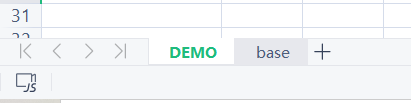base内容如下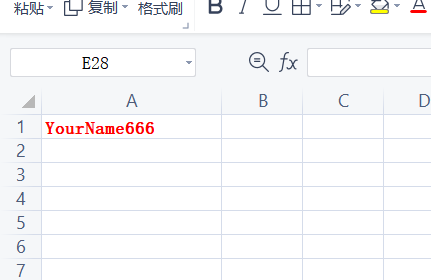DEMO内容如下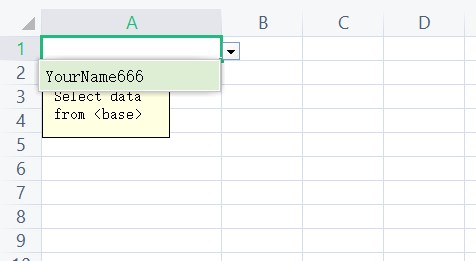展开全文python • C# Excel数据有效性 在Excel中，我们可以使用数据有效性功能来定义哪些数据可以被输入到工作表的单元格中，例如，限制输入的数据为一定范围内的数字或使用下拉列表来限制用户可选择的数据等。这篇文章主要分享如何... • EXCEL 2010从入门到精通》一、数据类型1、文本 输入数值型的文本数据，在前面先加一个单引号’ 例如：’1234567890 2、数字（数值） 输入分数：0 1/3（先输入0 + 空格，再输入分数） 3、日期、时间 / 或 - ... • 下面我想介绍的是如何设置数据有效性，以及对某个数据进行个数统计，具体我们通过以下两个例子详细说明一下。 设置数据有效性 我们经常用Excel设计测试用例，为了跟踪测试用例的状态，我们会对用例执行情况进行...测试用例 • 相信很多朋友都想通过Excel数据有效性实现多级联动下拉列表的制作，多级联动下拉列表就是第一列选择了某项，第二列则只会提供相对应的选项供我们选择，这个在网页中可以经常看到。 现在EXCEL也可以实现这样的效果... • 自定义注解导出excel数据 利用自定义注解方式，对数据列表进行简单的导出操作。即在实体对象的属性域上添加导出标识的注解，在对实体进行导出时，利用自定义注解进行反射的方法，获取实体需要导出的属性及值。该...注解 • 本章主要内容：分类汇总（分地区统计金额的总计，分地区与产品分类统计金额的总计，将分类汇总后的结果复制到其他区域），数据有效性 • 函数作用:18位身份证最后一位有效性验证............70 '49.函数作用:计算符合maturity condition的拆解金额....72 '50.函数作用:对多个用同一分隔符分隔的待查找元素，逐一在表区域首列内搜索，将返回选定单元格的... • 分类汇总就是把一些数据按照一个标准进行分类，然后按照相应的汇总方式进行汇总。 使用分类汇总之前先排序，否则汇总会出现很多类。 看如上这个表，如果按照所属区域分类，然后按照金额的总和汇总，在汇总之前... • npoi设置数据有效性 public void SetDataValidate(ISheet sheet, int firstCol, int lastCol) { CellRangeAddressList regions = new CellRangeAddressList(1, 65535, firstCol, lastCol); D... • ## Excel自定义格式详解 千次阅读 多人点赞 2018-07-19 11:54:59 因为参数的特殊，所以自定义的参数也是有关键字的。如函数=TEXT(A1,"b0000")就会显示错误。因为“b”就是保留的关键字，在自定义格式输入“b”系统就会自动填入“bb”。bb就是佛历年份，即以公元前543年为纪年元年...定义 详解 格式 • XSSFDataValidationConstraint（XSSF数据有效性验证约束）：创建约束的时候，可以指定数据类型，以及数据列。 例如： String strFormula = hiddenSheetName + "!$"+colStr+"$1:$"+colStr+"\$65535"; ...java poi
• 工作中往往会涉及到很多数据的处理，有些时候需要验证数据是否符合某个标准，比如学生的成绩，Excel中很好的给我们提供了检验数据的标准。...Excel数据无效的验证： 数据验证之圈释无效数据 自...
• 文章目录typora-copy-images-to: Excel Function Part IIEXCEL Function Part II Chapter 8 在数据有效性中使用公式认识数据有效性限定可以在单元格中输入的数据 EXCEL Function Part II Chapter 8 在数据有效...function
• 数据——有效性——自定义——公式——输入=or(len(a1)=15,len(a1)=18)，这样该单元格就只能输入15位或18位了。并可以输入错误的提示信息。 三、深入应用 这时要把整个E这个列设为该格式，全选这个E列，按照...
• 目录：【数据】–【有效性】 选项卡组成：【设置】【输入信息】【出错警告】【输入法模式】 方法：【设置】—允许：序列—来源：A,C, 这个比较常用，可以用辅助列，也可以不用辅助列。 1.2.2 自定义填充范围 效果： ...
• 因为数据有效性中不能直接引用别的工作表的内容，故而首先必须将sheet2的A列内容（假设在A1:A10中）定义为一个名称，然后设置sheet1 A2单元格的数据有效性，具体操作如下： 1.按ctrl+F3打开定义名称对话框，在...
• 在最近使用excel数据验证功能时，选择序列，字数的长度是有限制的数据库
• ## excel数据处理

千次阅读 多人点赞 2019-01-12 21:08:25
• 大家在使用Excel2007编辑工作表格时经常要输入大量数据，有时要求输入的这些数据是不允许重复的，例如常见... 　Step2：单击“数据”菜单，然后在“数据工具”功能区域中选择“数据有效性”选项，接下来在随工具
• Excel数据平台 是一款面向最终用户的管理软件系统设计工具与运行平台。 她将电子表格软件Microsoft Excel与关系型数据库系统MS SQL Server集成为一个网络数据业务协同工作环境。在这个平台上，用户通过设计模板、...
•  2、点击EXCEL的“数据”-“有效性”菜单，可弹出“数据有效性”设置窗口。  3、有效性有“整数”“小数”“序列”等共8种。  今天这里只讲解“序列”型，并且重点放在跨表引用上。 二、...